QUESTIONSHEET 1

(a) (i)    0.4 A                                                                                                                                               1                                       1

(ii)    0.4 A                                                                                                                                               1                                       1

(b) (i)    potential difference = current ´ resistance                                                                                          1                    1

2.4 V                                                                                                                                             1                                       1

(ii)    1.6 V                                                                                                                                               1                                       1

(c) showing all working                                                                                                                                  1                             1

correct answer with units for total resistance: 16 W                                                                                1               1

calculate third resistance: 6 W                                                                                                               1                           1

TOTAL  / 8

QUESTIONSHEET 2

(a) (i)    144 kJ / 144,000 J                                                                                                                             1                             1

(ii)   Lost in heating the surroundings, eg the kettle itself.                                                                            1               1

(b) (i)    10 A                                                                                                                                                1                                       1

(ii)    iron / cooker / covector heater /

any appliance whose main purpose is producing heat                                                                           2

(c) (i)    2.4 ´ 20/10 = 4.80                                                                                                                             1                                  1

(ii)    0.1 ´ 20 ´ 10 = 20p                                                                                                                           1                                       1

(iii)     20    ´  100 = 4.17%                                                                                                                       1                                  1

480

TOTAL  / 8

QUESTIONSHEET 3

(a) time = 5 ´ 3 minutes = 15 minutes = 1/4 hour                                                                                             1
units = 2.4 kW ´ 1/4 hour = 0.6 kWh                                         1
cost = 0.6 ´ 8p = 4.8p                                                                                                                               1

(b) (i)     Power = current x voltage                                                                                                                 1

(ii)    current = power/voltage = 2400 W/240 V                                                                                           1
10 A                                                                                                                                                1

(iii)   13 A                                                                                                                                                1

(c) If the cable/cord was damaged could be a large current                                                                              1

TOTAL  / 8

QUESTIONSHEET 4

(a) (i)           12V - 10V = 2 V                                                                                                                        1

(ii)     Voltage  = current ´ resistance                                                                                                        1

(iii)     2 V/1000 W                                                                         1
0.002 A or 2 mA                                                                                                                             1

(b) (i)      0.008 A ´ 1000 W                                                                                                                           1
8V                                                                                                                                                 1

12 - 8 = 4 V                                                                                                                                    1

(ii)    4 V/0.008 A                                                                                                                                   1

= 500 W                                                                                                                                         1

TOTAL  / 9

QUESTIONSHEET 5

(a) dangerous                                                                                                                                                1
causing much heat and possibly a fire                                                                                                        1

(b) If  fault develops circuit breaker acts more quickly than a fuse                                                                    1
reducing the chance of electrocution.                                                                                                         1

TOTAL  / 5

QUESTIONSHEET 6

(a) (i)    High heat

parallel connection (A to B & A to D)                                                                                                1                    1

no connection C to D                                                                                                                        1                             1

Medium heat

Single connection A to B                                                                                                                   1                           1

No connection C to D                                                                                                                       1                             1

Low heat

Connection A to B                                                                                                                            1                             1

And C to D                                                                                                                                      1                                  1

(ii)    Parallel circuits each element                                                                                                             1                           1

has same current                                                                                                                              1                             1

medium heat has only one element                                                                                                     1                         1

therefore half the heat                                                                                                                       1                             1

series circuit shares current                                                                                                               1                           1

between elements                                                                                                                             1                             1

TOTAL  / 12

QUESTIONSHEET 7

(a) (i)    3000 / 240                                                                                                                                        1                                  1

=  12.5 amps                                                                                                                                     1                                  1

(ii)    13 amps                                                                                                                                           1                                  1

(b) 240 / 12.5                                                                                                                                                 1                                  1

=  19.2 ohms                                                                                                                                            1                                  1

(c) earth connected to case of fire                                                                                                                  1                         1

TOTAL  / 6

QUESTIONSHEET 8

(a) 5A ´ 240 V                                                                                                                                                  1                             1

=  1200 W                                                                                                                                                                                   1

1200 / 60                                                                                                                                                      1                                  1

=  20                                                                                                                                                            1                      1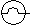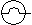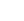(b)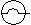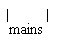3

(c) 6 ´ 15 ´ 1.2 = total units                                                                                                                           1                             1

= 108 kWh                                                                                                                                                                           1

Price  = 108 ´ 12 p                                                                                                                                  1                             1

= £12.96                                                                                                                                                  1

TOTAL  / 10

QUESTIONSHEET 9

(a) voltage across coil 1  =  number of turns in coil 1voltage across coil 2      number of turns in coil 2                                                                                   1

working                                                                                                                                                   1                                       1

correct answer with units:  12 V                                                                                                                1                         1

(b) (i)    current / electric current / current flow                                                                                               1                    1

(ii)    A2                                                                                                                                                   1                                  1

(c) (i)    low current                                                                                                                                       1                                  1

(ii)    1 mark for answers which include the idea that:

flow of current converts some electrical energy to heat energy which is lost by convection                    1                                  1

1 mark for answers which include the idea that:

transmitting at high voltage is more efficient than at high current.                                                         1

TOTAL  / 8

QUESTIONSHEET 10

(a) (i)    a resistor whose resistance changes with temperature.                                                                        1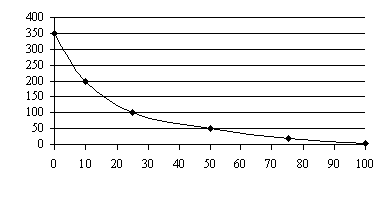(ii)

 Resistance (kW)

 Temperature (oC)

Deduct 1 mark for each incorrectly plotted point to a maximum of 2.                                                    2                                       2

(iii)   decreases / goes down                                                                                                                      1                             1

(b) (i)    decreases / goes down                                                                                                                      1                             1

(ii)    potential difference = current ´ resistance                                                                                          1                    1

0.04 mA / 0.00004 A                                                                                                                         1                             1

(c) Light dependent resistor (LDR)                                                                                                                 1                         1

TOTAL  / 8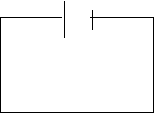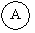QUESTIONSHEET 11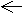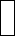(a) all components present:  battery or cell,

variable resistor, bulb and ammeter all in series,                                                                                          1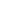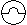voltmeter connected in parallel with bulb only.                                                                                            1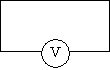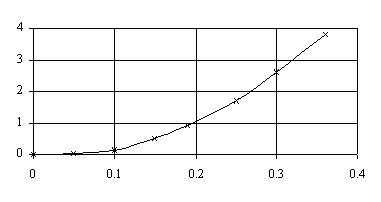(b) (i)

 Potential Difference (V)

 Current (A)

Deduct 1 mark for each incorrectly plotted point to a

maximum of 2.                                                                                                                                  2                             2

(ii)    1 V  + 0.1 V                                                                                                                                     1                                  1

(iii)   resistance = potential difference

current                                                                                                                1                           1

5 W  + 0.5 W                                                                                                                                    1                                  1

(iv)   increases                                                                                                                                          1                                  1

TOTAL  / 8

QUESTIONSHEET 12

(a) (i)     787, 748, 604, 527:

all correct                                                                                                                                         2                                  2

3 correct.                                                                                                                                         1                                  1

(ii)    December 1998                                                                                                                                1                                  1

(iii)   Weather colder so more energy required for heating or cooking/

nights longer so more lighting required.                                                                                              1                    1

(b) (i)    times (h): 0.25, 0.25, 2, 0.5, 4                                                                                                             1                           1

kWh: 0.5, 0.175, 0.4, 0.4, 0.41                                                                                                            1                           1

Deduct 1 mark per incorrect answer to a total of 3 marks.

(ii)    18.75p                                                                                                                                              1                                       1

TOTAL  / 8

QUESTIONSHEET 13

(a) low energy bulb                                                                                                                                        1

(b) units = 0.15 kW ´ 1000 = 150 units

(note the change of watt to kilowatt)                                            1
150 ´ 8p                                                                                       1
1200 p or £12                                                                                                                                           1

(c) £12 ¸ 5 = £2.40                                                                                                                                       1

TOTAL  / 5

QUESTIONSHEET 14

(a) 1000 W (power is measured in watt, W)                                                                                                    1

(b) (i)     Power = current ´ voltage                                                                                                                1

(ii)    current = power/voltage = 1000 W/230 V                                 1
4.3(5) A                                                                                                                                           1

(c) (i)    safety device                                                                              1
if too much current flows, it breaks and prevents

further current flow                                                                                                                         1

(ii)   5 A (use the lowest value which will allow the device to operate normally.)                                          1

TOTAL  / 7

QUESTIONSHEET 15

(a) 1100 W                                                                                                                                                    1                                  1

(b) (i)     Power = current ´ voltage                                                                                                               1

(ii)     current = power/voltage = 1100 W/220 V                                                                                         1
5 A                                                                                                                                                1

(c) (i)    Voltage = current ´ resistance                                                                                                           1

(ii)    resistance = voltage/current = 220 V/5 A                                                                                           1
44 W                                                                                                                                                1

(d) Assume the heating element has the same resistance
current = voltage/resistance = 110 V/44 W = 2.5 A                                                                                     1
power = current ´ voltage = 2.5 A ´ 110 V = 275 W                                                                                  1
new power = 275/1100 = 1/4 of original power                                                                                           1

TOTAL  / 10

QUESTIONSHEET 16

(a) if a short circuit occurs                                                                                                                             1                           1

current will flow to earth                                                                                                                           1                           1

case will not become live                                                                                                                          1                           1

(b) short circuit causes fuse to heat up                                                                                                            1                         1

and melt                                                                                                                                                   1                                  1

cutting off power                                                                                                                                      1

TOTAL  / 6

QUESTIONSHEET 17

(a) current = power/voltage = 3000 W/240 V                                                                                                  1
12.5 A                                                                                                                                                     1

(b)

 wire current /A earth 0 live 12.5 neutral 12.5

3

(c) Very large current flows                                                                                                                           1
in the live and earth wires                                                                                                                         1
fuse melts and breaks, preventing further current flow.                                                                               1

TOTAL  / 8

QUESTIONSHEET 18

(a)

 Wire Name Colour A NEUTRAL BLUE B EARTH GREEN AND YELLOW C LIVE BROWN

3                           3

(b) (i)       earth wire                                                                                                                                      1                                       1

(ii)           since the case of the hairdryer is plastic, there are no exposed metal parts                                     1                             1
no danger of electrocution if the live wire touches the plastic case.                                                     1

1

(c) Fuse protects wires                                                                                                                                  1                                  1

high current flows & melts fuse wire                                                                                                         1                         1

circuit breaks                                                                                                                                           1                                  1

TOTAL  / 9

QUESTIONSHEET 19

(a) kilowatt                                                                                                                                                    1                                  1

one hour                                                                                                                                                  1                                  1

unit                                                                                                                                                          1                                       1

(b) 3 600 000                                                                                                                                                1                         1

3.6 mV                                                                                                                                                                                      1

(c) (i)    3 amp                                                                                                                                                                                       1

13 amp                                                                                                                                                                                     1

5 amp                                                                                                                                                                                       1

(ii)    A    3 ´ 2 = 6 units                                                                                                                            1                             1

B    0.1 ´ 12 = 1.2 units                                                                                                                     1                           1

Immersion heater                                                                                                                              1                             1

TOTAL  / 11

QUESTIONSHEET 20

One mark for each of

current

fire

damaged

metals

melting

5 amps

melt

live

TOTAL  / 8